# CBSE Class 12 Economics Income and Employment Revision Notes

Key concepts

• • Aggregate demand and its components.
• • Consumption function and Saving Function
• • Propensity to consume and propensity to save
• • Short run equilibrium Output Equilibrium level of income and output is determined where,1) AD=AS,  2) Planned saving =planned investment (S=I).
• • Investment multiplier and the multiplier mechanism.
• • Meaning of full employment and involuntary unemployment.
• • Problems of excess demand and deficient demand.
• • Measures to correct excess demand and deficient demand
• • Aggregate demand in macroeconomics

It refers to sum total of expenditure on the domestically produced goods and services during the period of an accounting year.

Four Components of Aggregate Demand

•  Private consumption expenditure ( C ) it refers to total expenditure incurred by all household on the purchase of goods & services during an accounting year.
•  Investment expenditure(I) - It refers to total expenditure incurred by all the private firms or capital goods.
•  Government Expenditure ( G) It refers to total expenditure incurred by govt. on consumer goods & capital goods.
• • Net Export–It means Export – Import (X-M)

AD = C+ I+ G+ (X- M)

• Consumption Function-It expresses functional relationship between aggregate consumption and national income.  C= f ( Y )

Consumption function depends on Propensity to consume.

It is of two types:

1.Average Propensity to Consume (APC )   APC= C /Y

2. Marginal Propensity to Consume (MPC )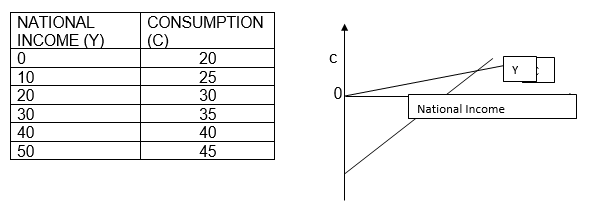i) At lower level of income ,consumption is higher than income because there is  always same minimum level of consumption expenditure required even at 0 level of income. (past saving or borrowing). C > Y (Minimum level of consumption)=autonomous consumption.

ii) After a point income is equal to consumption this point is called break-even point. C=Y .

iii)  As income increases consumption also increases but lower than the rate of increase in income .  C< Y

MPC is the ratio of change in consumption(C) to change in income(Y) is known as Marginal Propensity to Consume. It indicates the proportion of additional income that is being spent on consumption.

MPC=∆C/∆Y

MPC+MPS=1

Aggregate Investment is of two types:-

Autonomous Investment : It is that expenditure on capital formation which is undertaken independently of the level of income.

Induced Investment: It is directly related with the level of income.

Aggregate supply is the total amount of money value of goods and services that all the producers are willing to supply in an economy in a year.

In fact , it refers to the national income of a country.

Aggregate Supply = National Income

Aggregate Supply (AS ) = Consumption ( C ) + Saving ( S)

AS= C + S

Saving Function-It shows the functional relationship between savings and income. S=f(Y)

S= -C  + (1-b)Y    ( as we know S=Y-C)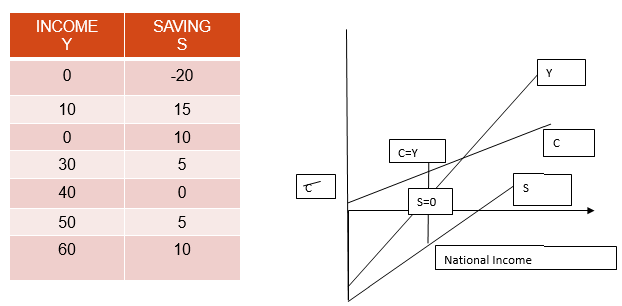Determination of equilibrium level of income

Two Approaches

As we know that equilibrium level of national income is determined under the given condition of aggregate demand and aggregate supply, and has tendency to stick to that level i.e., where aggregate demand is equal to aggregate supply. AD=AS

When aggregate demand falls short off aggregate supply, than national income will decrease.

When AD<AS, then there would be stockpiling and producers will produce less. National income will fall and as we know positive relationship exists between national income and consumption, so consumption will fall, which will thereby decrease the aggregate demand till we reach the equilibrium.

S = I

The economy achieves equilibrium level of income is achieved when S=I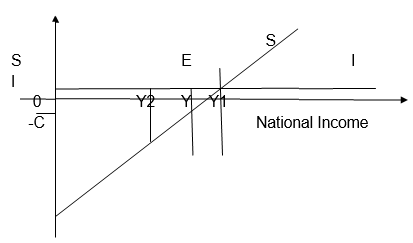In the above figure, the equilibrium level of national income is attained at point E, when savings= investment which is derived from a point where S=I.

If due to some disturbance we divert from our position like when investment>savings (at Y2), then production will have to be increased to meet the excess demand. Consequently, national income will increase leading to rise in saving until saving becomes equal to investment. It is here that equilibrium level of income is established because what the savers intend to save becomes equal to what the investors intend to invest.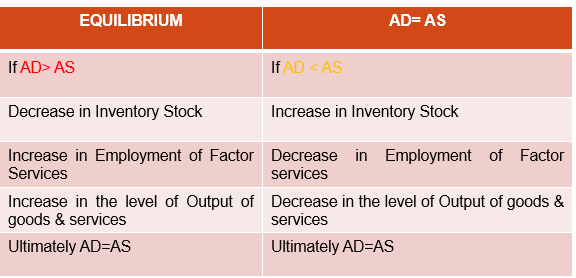Full employment equilibrium refers to the situation where aggregate demand is equals to aggregate supply and all those who are able to work and willing to work (at the existing wage rate) are getting work.

Over full employment refers to the situation when equilibrium is attained , that is aggregate demand is equal to aggregate supply beyond the full employment level.

Excess Demand / Inflationary Gap

When economy aggregate demand exceeds “aggregate supply at full employment level”, the demand is said to be an excess demand.

When in an economy aggregate demand exceeds ‘aggregate supply at full employment level’, the demand is said to be excess demand and the gap is called inflationary gap.

Causes

1. Increase in household consumption demand due to rise in propensity to consume.

2. Increase in private investment demand because of rise in credit facilities.

3. Increase in public (government) expenditure.

4. Increase in export demand.

5. Increase in money supply or increase in disposable income Impacts

Effect on general price level

A rise to general price level.

Effect on output

No effect on the level of output.

Effect on employment

No change in the level of employment.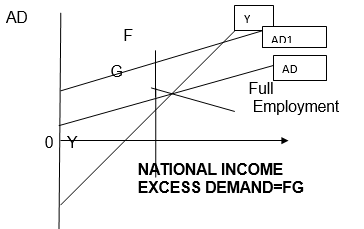• Deficient Demand/ Deflationary Gap When in an economy aggregate demand falls short of aggregate supply at full employment level the demand is said to be deficient demand and the gap is called deflationary gap.

• Causes -Decrease in household consumption demand due to fall in propensity to consume.

• Decrease in private investment demand because of fall in credit facilities.

• Decrease in public (government) expenditure.

• Decrease in export demand.

• Decrease in money supply or decrease in disposable income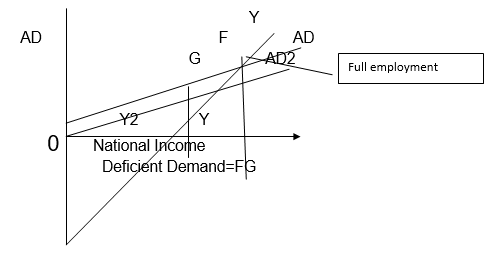Impact

Effect on general price level .A fall to general price level.

Effect on output Low effect on the level of output.

• Effect on employment  Involuntary unemployment.

• Measures to correct Excess Demand and Deficient Demand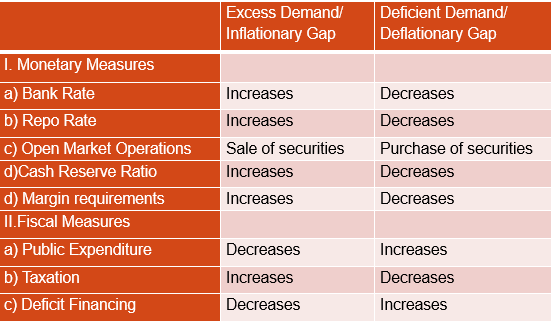Numerical Examples

Formulae

APC=C/Y

APS= S/Y

APC+APS=1

MPC= ΔC/ΔY

MPS= ΔS/Δ Y

MPC+MPS=1

K=   ΔY /Δ I

K= 1/ 1-MPC

K= 1/ MPS

C=  C + b Y

Complete the following table: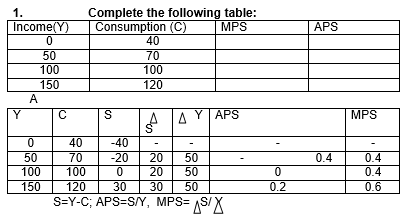Using the equation of consumption function: C= C  + b Y, calculate consumption expenditure at the income level Rs 1000 crore, if autonomous consumption is Rs 20 crore and 20% of additional income is saved.A given MPS= 0.2 ( as20% of additional income is saved) MPC= 0.8 (=1-MPS)

C= 20 + 0.8 x 1000= Rs 820 crore With the help of saving function S= -50 + 0.2 Y. calculate consumption expenditure at the income level of Rs 5000 crore.

S=  -  C    + (1-b) Y

C = 50 + 0.8 x 5000= Rs. 4050 crore

## Tags:

Click for more Economics Study Material
 CBSE Class 12 Economics Forms of Market Revision Notes CBSE Class 12 Economics National Income Revision Notes CBSE Class 12 Economics Money and Banking Revision Notes CBSE Class 12 Economics 2015 examination guidance CBSE Class 12 Economics Determination of Income and Employment Revision Notes CBSE Class 12 Economics Introduction Revision Notes CBSE Class 12 Economics Income and Employment Revision Notes CBSE Class 12 Economics Consumers Equilibrium and Demand Revision Notes CBSE Class 12 Economics Balance of Payment Revision Notes CBSE Class 12 Economics Producer Behaviour and Supply Revision Notes

## Latest NCERT & CBSE News

Read the latest news and announcements from NCERT and CBSE below. Important updates relating to your studies which will help you to keep yourself updated with latest happenings in school level education. Keep yourself updated with all latest news and also read articles from teachers which will help you to improve your studies, increase motivation level and promote faster learning

### CBSE OMR Sheet Guidelines for Term 1

You are aware that the CBSE will be using OMR for the first time for assessment of both the Classes-X and XII in Term-I examinations. Hence, there is a need that all the students appearing in the Term-l examinations and the schools sponsoring these students should have...